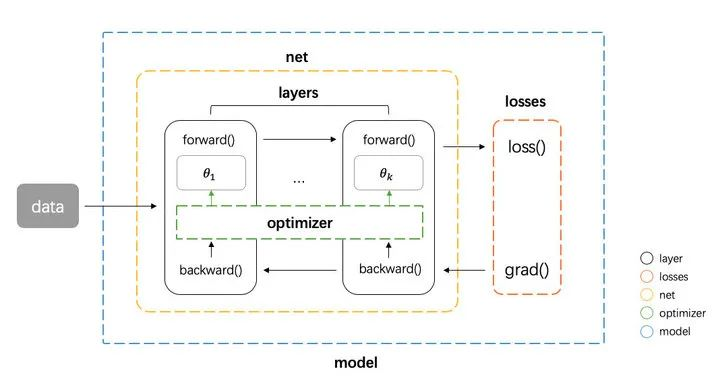2022年03月02日

## 抽象层

### 组件抽象

1. 数据在网络层之间的流动：前向传播和反向传播可以看做是张量 Tensor（多维数组）在网络层之间的流动（前向传播流动的是输入输出，反向传播流动的是梯度），每个网络层会进行一定的运算，然后将结果输入给下一层
2. 计算损失：衔接前向和反向传播的中间过程，定义了模型的输出与真实值之间的差异，用来后续提供反向传播所需的信息
3. 参数更新：使用计算得到的梯度对网络参数进行更新的一类计算

1. tensor 张量，这个是神经网络中数据的基本单位
2. layer 网络层，负责接收上一层的输入，进行该层的运算，将结果输出给下一层，由于 tensor 的流动有前向和反向两个方向，因此对于每种类型网络层我们都需要同时实现 forward 和 backward 两种运算
3. loss 损失，在给定模型预测值与真实值之后，该组件输出损失值以及关于最后一层的梯度（用于梯度回传）
4. optimizer 优化器，负责使用梯度更新模型的参数 然后我们还需要一些组件把上面这个 4 种基本组件整合到一起，形成一个 pipeline

5. net 组件负责管理 tensor 在 layers 之间的前向和反向传播，同时能提供获取参数、设置参数、获取梯度的接口
6. model 组件负责整合所有组件，形成整个 pipeline。即 net 组件进行前向传播 -> losses 组件计算损失和梯度 -> net 组件将梯度反向传播 -> optimizer 组件将梯度更新到参数。### 组件实现

``````# define model
net = Net([layer1, layer2, ...])
model = Model(net, loss_fn, optimizer)
# training，将 net、loss、optimizer 一起传给 model，model 实现了 forward、backward 和 apply_grad 三个接口分别对应前向传播、反向传播和参数更新三个功能
pred = model.forward(train_X)
# inference
test_pred = model.forward(test_X)
``````

tensor 张量是神经网络中基本的数据单位，我们这里直接使用 numpy.ndarray 类作为 tensor 类的实现。 layer需要有提供 forward 和 backward 接口进行对应的运算。同时还应该将该层的参数和梯度记录下来。先实现一个基类如下

``````class Layer(object):
def __init__(self, name):
self.name = name
def forward(self, inputs):
raise NotImplementedError
raise NotImplementedError
``````

``````class Dense(Layer):
def __init__(self, num_in, num_out,w_init=XavierUniformInit(),b_init=ZerosInit()):
super().__init__("Linear")
self.params = {
"w": w_init([num_in, num_out]),
"b": b_init([1, num_out])}
self.inputs = None
# forward 方法接收上层的输入 inputs，实现  的运算
def forward(self, inputs):
self.inputs = inputs
return inputs @ self.params["w"] + self.params["b"]
# backward 的方法接收来自上层的梯度，计算关于参数  和输入的梯度，然后返回关于输入的梯度
``````

``````class Activation(Layer):
"""Base activation layer"""
def __init__(self, name):
super().__init__(name)
self.inputs = None
def forward(self, inputs):
self.inputs = inputs
return self.func(inputs)
def func(self, x):
raise NotImplementedError
def derivative_func(self, x):
raise NotImplementedError
``````

net 类负责管理 tensor 在 layers 之间的前向和反向传播

``````class Net(object):
def __init__(self, layers):
self.layers = layers
# 按顺序遍历所有层，每层计算的输出作为下一层的输入
def forward(self, inputs):
for layer in self.layers:
inputs = layer.forward(inputs)
return inputs
# 逆序遍历所有层，将每层的梯度作为下一层的输入
for layer in reversed(self.layers):

for layer in self.layers:
def get_parameters(self):
return [layer.params for layer in self.layers]
def set_parameters(self, params):
for i, layer in enumerate(self.layers):
for key in layer.params.keys():
layer.params[key] = params[i][key]
``````

losses 组件需要做两件事情

``````class BaseLoss(object):
# 计算损失值
def loss(self, predicted, actual):
raise NotImplementedError
# 计算损失值和关于预测值的梯度
raise NotImplementedError
``````

optimizer 主要实现一个接口 compute_step，这个方法根据当前的梯度，计算返回实际优化时每个参数改变的步长。

``````class BaseOptimizer(object):
def __init__(self, lr, weight_decay):
self.lr = lr
self.weight_decay = weight_decay
step = list()
# compute step
p = 0
for param in params:
layer = dict()
for k, v in param.items():
block = np.prod(v.shape)
_step = flatten_step[p:p+block].reshape(v.shape)
_step -= self.weight_decay * v
layer[k] = _step
p += block
step.append(layer)
return step

raise NotImplementedError
``````

``````class Model(object):
def __init__(self, net, loss, optimizer):
self.net = net
self.loss = loss
self.optimizer = optimizer

def forward(self, inputs):
return self.net.forward(inputs)

def backward(self, preds, targets):
loss = self.loss.loss(preds, targets)
params = self.net.get_parameters()
return loss, step

for k, v in param.items():
``````

## 执行层

### 极简demo

``````#include <iostream>
#include <iomanip>
#include <string>
#include <unordered_map>
#include <random>
#include <chrono>
#include <any>
//自定义 Tensor 类型，这里数据成员非常简单，就是个标量，重载了基本数学运算符
class MyTensor {
public:
uint32_t data;
public:
MyTensor(){};
MyTensor(uint32_t x) : data(x) {}
MyTensor operator*(const MyTensor& a) {
this->data = this->data * a.data;
return *this;
}
MyTensor operator+(const MyTensor& a) {
this->data = this->data + a.data;
return *this;
}
MyTensor operator-(const MyTensor& a) {
this->data = this->data - a.data;
return *this;
}
MyTensor operator*(const int& a) {
this->data = this->data * a;
return *this;
}
};

// Op 基类
class OpBase {
public:
std::unordered_map<std::string, MyTensor> inputs;
std::unordered_map<std::string, MyTensor> outputs;
std::unordered_map<std::string, MyTensor> labels;
public:
virtual void Run() = 0;
};

// 乘法前向 Op
class MultipylyForward : public OpBase {
public:
void Run() {
MyTensor x = inputs["X"];
MyTensor w = inputs["W"];
MyTensor y1 = x * w;
outputs["Y"] = y1;
}
};

// 乘法反向 Op
class MultipylyBackward : public OpBase {
public:
void Run() {
MyTensor x = inputs["X"];
outputs["Y"] = x;
}
};

// 加法前向 Op
class AddForward : public OpBase {
public:
void Run() {
MyTensor x1 = inputs["X1"];
MyTensor x2 = inputs["X2"];
MyTensor y = x1 + x2;
outputs["Y"] = y;
}
};

// 加法反向 Op
class AddBackward : public OpBase {
public:
void Run() {
MyTensor x;
x.data = 1;
outputs["Y"] = x;
}
};

// loss 前向 Op，这里选取 MSE 作为示例
class LossForward : public OpBase {
public:
void Run() {
MyTensor y = inputs["X"];
MyTensor label = labels["Label"];
MyTensor loss = (y - label) * （y - label）;
outputs["Y"] = loss;
}
};

// loss 反向 Op
class LossBackward : public OpBase {
public:
void Run() {
MyTensor y = inputs["X"];
MyTensor label = labels["Label"];
outputs["Y"] = (y - label) + (y - label);
}
};

// 梯度更新 Op
class UpdateGrad : public OpBase {
public:
double lr = 0.1;
std::unordered_map<std::string, MyTensor> inputs;
std::unordered_map<std::string, MyTensor> outputs;
public:
void Run() {
MyTensor w = inputs["W"];
MyTensor lr;
lr.data = this->lr;
outputs["Y"] = w - lr * grad;
}
};

int main() {
//1. 用户自定义前向组网

//2. 框架生成前向op + 自动补全反向OP + 插入梯度更新op

//3. 实例化 c++ 端 op 对象
std::vector<OpBase*> opClass {new MultipylyForward(), new AddForward(), new LossForward(),

//4. 框架根据用户组网，自动给每个op的输入赋值，这里仅以乘法前向op作个例子。一定要记住一点：框架中所有输入数据、
//参数、模型中间输入、输出、以及每个参数的梯度都有一个 string 类型的名字，它的存在是为了给op输入赋值服务的
opClass->inputs["X"] = MyTensor(10);
opClass->inputs["W"] = MyTensor(20);
for (auto op : opClass) {
op->Run();
}

//5. 测试第1个op的输出
std::cout << opClass->outputs["Y"].data;  // 输出结果：200
}
``````

## 推荐文章

1. BIDMach的整体设计分三层，下层关注于底层硬件的性能并统一封装为矩阵运算和Actor间的通信操作；中间层是各种机器学习算法的计算图封装，而最上层则是面向用户设计的交互式机器学习工具。
2. 一般来说，类似tensorflow/pytorch这样的深度学习框架，实际上是提供了一个可微计算图引擎，他们可以非常方便地构建一个可微的函数（如下图所示），然后基于数据去最小化损失函数，这种抽象使得深度学习变得非常简单和可行易用。但是到了工业界(搜索/推荐/广告)，实际的机器学习问题远不止这么简单，我们要重新思考一下框架的职责。框架是只需要专注于做损失函数优化就可以呢？还是会有很多其它事情也要去负责呢？
1. 比如图中所示的x、y样本数据本身的生成就是一个很大的问题：比如样例生成或者特征萃取。而且在互联网应用中，我们往往需要从流式的数据流中实时地完成x、y的生成。
2. 到了工业界中，训练和推理很可能是要被分开去做的。因为在推理的时候你只需要处理一个固定的计算图，这就存在很多优化的空间。

所以实际情况是，如果把框架的概念从一个单点应用扩展到一个可用的工业界框架后，就会包含很多模块：样本的处理、特征的处理、离线训练和在线推理，各种数据接口，一致性保障、资源管理和整个实验平台等等一系列工具。这些东西可能从广义来讲都算是框架中的一部分。

3. 框架与算法共同进化。工程框架的发展是跟整个算法的红利包括跟整个业务的发展都有关联的。算法侧提出了一个新的结构，框架就需要去做适配和推理优化。框架的革新又会导致算法工程师可以去尝试更复杂更加有意思且更有深度的想法。整个过程就是一个共同进化共同演化的过程。

1. 输入的改造，开源的实现的输入格式和特征构造通常和线上不一致，适配一个算法通常需要1-2周左右的时间，还难免因为对代码的不熟悉引入bug，如果要尝试5个算法的话，就需要5倍的改造时间。如果算法资源有限，这时候是不是就要忍痛割爱，放弃一些可能有效果的尝试了？
2. 开源的实现很多只是在公开数据集上取得了比较好的效果，在公开数据集上的最优参数也不一定适合实际的场景，因此参数调优也需要较大的工作量；如果没有系统化的调参方法，很多算法也就是简单试一下，没有deep explore，哪来对算法的深入理解呢? 为什么看似简单的改进，你没有能够发现呢？
3. 开源的实现用的是TensorFlow 1.4，而线上用的TensorFlow 2.3，好多函数的参数都变掉了
4. 费了九牛二虎之力把模型效果调好了，发现上线也会有很多问题，比如训练速度太慢、内存占用太大、推理qps跟不上、离线效果好在线效果跪等等。